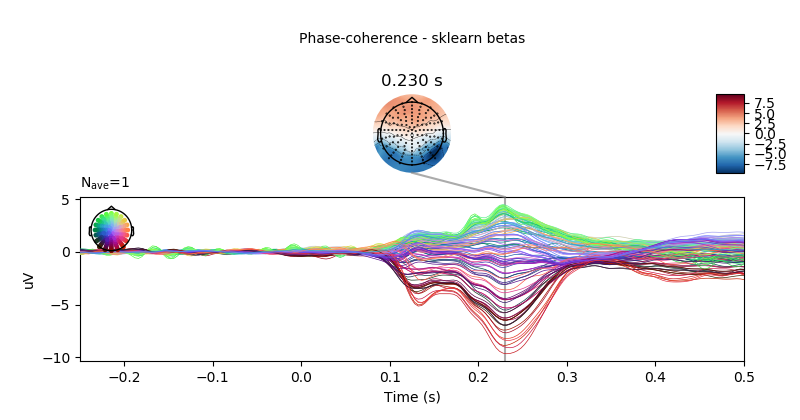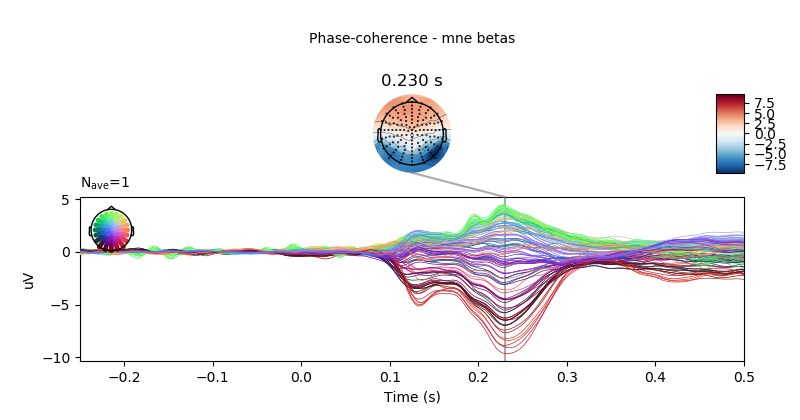# Plot beta coefficients from linear model estimation with sklearn¶

```# Authors: Jose C. Garcia Alanis <alanis.jcg@gmail.com>
#

import numpy as np
from sklearn.linear_model import LinearRegression

from mne.decoding import Vectorizer, get_coef
from mne.datasets import limo
from mne.stats import linear_regression
from mne.evoked import EvokedArray
```

Here, we’ll import only one subject and use the data to fit linear regression using LinearRegression from sklearn.linear_model. We’ll compare this results of the output of mne.stats.linear_regression

```# subject id
subjects = 
# create a dictionary containing participants data
limo_epochs = {str(subj): limo.load_data(subject=subj) for subj in subjects}

# interpolate missing channels
for subject in limo_epochs.values():

# epochs to use for analysis
epochs = limo_epochs['2']

# only keep eeg channels
epochs = epochs.pick_types(eeg=True)

# save epochs information (needed for creating a homologous
# epochs object containing linear regression result)
epochs_info = epochs.info
tmin = epochs.tmin
```

Out:

```1052 matching events found
No baseline correction applied
0 projection items activated
Computing interpolation matrix from 117 sensor positions
Interpolating 11 sensors
```

use epochs metadata to create design matrix for linear regression analyses

```# add intercept
# effect code contrast for categorical variable (i.e., condition a vs. b)
design['face a - face b'] = np.where(design['face'] == 'A', 1, -1)
# create design matrix with named predictors
predictors = ['intercept', 'face a - face b', 'phase-coherence']
design = design[predictors]
```

extract the data that will be used in the analyses

```# get epochs data
data = epochs.get_data()

# number of epochs in data set
n_epochs = data.shape

# number of channels and number of time points in each epoch
# we'll use this information later to bring the results of the
# the linear regression algorithm into an eeg-like format
# (i.e., channels x times points)
n_channels = data.shape
n_times = len(epochs.times)

# vectorize (channel) data for linear regression
Y = Vectorizer().fit_transform(data)
```

fit linear model with sklearn

```# here, we already have an intercept column in the design matrix,
# thus we'll call LinearRegression with fit_intercept=False
linear_model = LinearRegression(fit_intercept=False)
linear_model.fit(design, Y)

# next, we'll extract the resulting coefficients (i.e., betas)
# from the linear model estimator.
betas = get_coef(linear_model, 'coef_')

# notice that the resulting matrix of coefficients has a shape of
# number of observations in the vertorized channel data (i.e, these represent
# teh data points want to predict) by number of predictors.
print(betas.shape)

# thus, we can loop through the columns (i.e., the predictors) of the
# coefficient matrix and extract coefficients for each predictor and project
# them back to a channels x time points space.
lm_betas = dict()
for ind, predictor in enumerate(predictors):
# extract coefficients
beta = betas[:, ind]
# back projection to channels x time points
beta = beta.reshape((n_channels, n_times))
# create evoked object containing the back projected coefficients
# for each predictor
lm_betas[predictor] = EvokedArray(beta, epochs_info, tmin)
```

Out:

```(25728, 3)
```

plot results of linear regression

```# only show -250 to 500 ms
ts_args = dict(xlim=(-.25, 0.5))

# visualise effect of phase-coherence for sklearn estimation method.
lm_betas['phase-coherence'].plot_joint(ts_args=ts_args,
title='Phase-coherence - sklearn betas',
times=[.23])
```replicate analysis using mne.stats.linear_regression

```reg = linear_regression(limo_epochs['2'], design, names=predictors)

# visualise effect of phase-coherence for mne.stats method.
reg['phase-coherence'].beta.plot_joint(ts_args=ts_args,
title='Phase-coherence - mne betas',
times=[.23])
```Out:

```Fitting linear model to epochs, (25728 targets, 3 regressors)
Done
```

Total running time of the script: ( 0 minutes 4.110 seconds)

Gallery generated by Sphinx-Gallery